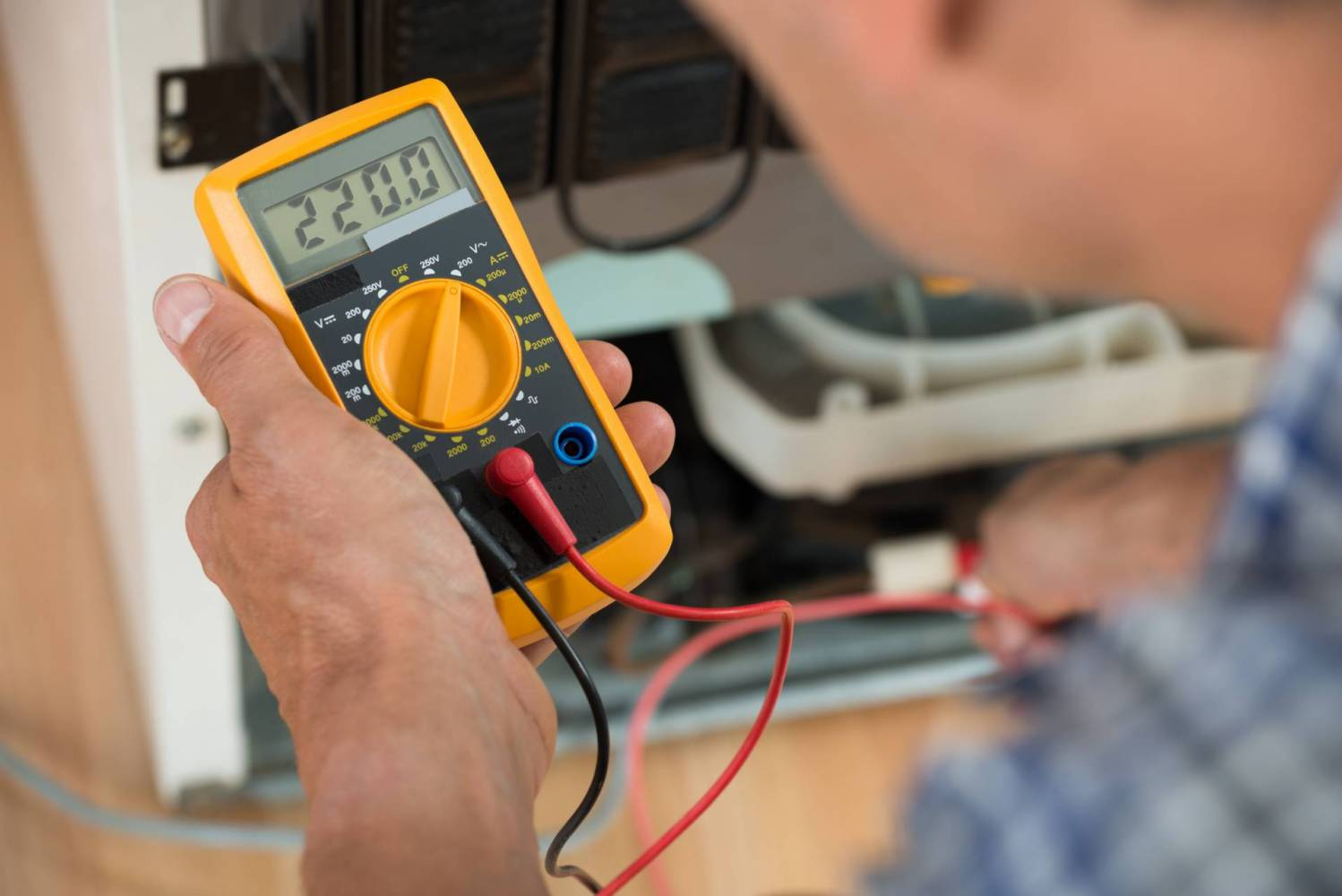What is a Joule?Do You Know What a Joule Is?

Understanding measurement units like joules is key to understanding how electricity works. While we’re not going to get deep in the weeds on math and physics here, we will offer a brief guide to joules, watts, and volts. Here are the basics.

What Is a Joule?

Pronounced “jewel,” a joule is a measure of energy used in the International System of Units. It is named after the English physicist James Prescott Joule, who developed the first law of thermodynamics. Joule also developed the Kelvin scale with his colleague William Thomson.

A joule is equal to the energy transferred to an object when a single force of newton acts on it. It is also a measurement of the energy given off when an electrical current of one amp passes through a resistance of one ohm for one second.

What Does That Mean in Real Terms?

A joule is equivalent to:

• The amount of electricity needed to run a 1-watt device for one second.
• The energy needed to lift a medium-sized tomato is 1 meter (3 foot 3 inches).
• The heat required to raise the temperature of water from 0 degrees Celsius to 1 degree, or from 32 degrees Fahrenheit to 33.8.
• The amount of energy released as heat by an average person at rest every 1/60 of a second.
• The kinetic energy of a 50 kg or 110-pound human moving very slowly.
• The food energy in half of a sugar crystal.

Calculating Volts to Joules

To calculate volts into joules, you must first know the coulombs of the electrical charge it uses. A coulomb is a unit of electrical charge that’s equal to the quantity of electricity conveyed in one second by a current of one amp.

The formula for calculating the Volts (V) to Joules (J) E (J) = V (V) x Q (C).

Example: If the voltage supply of an electrical circuit is 65 V and the charge flow is 10 Coulombs, what is the energy in joules?

E (J) = V (V) x Q (C) = 65 V x 10 C = 650 Joules (J)

What Is Equivalent To a Joule in Electrical Terms?

A watt-second is a derived unit of energy equivalent to the joule. The watt-second is the power of one watt sustained for one second. The symbol for watt-second is W or w-s.

How Are Joules Different from Watts?

A watt is a measure of power. It measures how fast energy is used, which is also known as energy over time. A joule is a measure of energy.

How To Convert Watts to Joules

Do you want to know how much energy a 60-watt bulb uses in 120 seconds?

To convert watts to joules, multiply the number of watts by the number of seconds the current runs. A 1-watt device consumes 1 joule of energy every second.

In this example, 60 watts X 120 = 7200. A 60-watt bulb uses 7200 joules in 120 seconds.

You Can Count on SESCOS

You don’t need advanced knowledge of electricity to deal with electrical problems in your home or business. SESCOS can take care of all your electrical needs. If you need help, call us today.

24/Hour Emergency Electricians | 703-777-6200